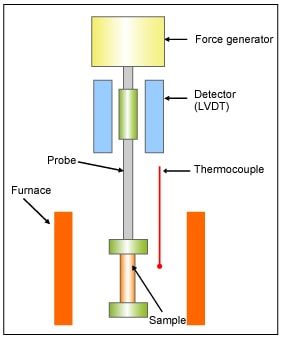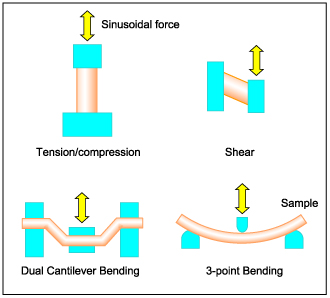# Principle of Dynamic Mechanical Analysis (DMA)

## Definitions of Dynamic Mechanical Analysis (DMA)

• A technique in which the sample's kinetic properties are analyzed by measuring the strain or stress that is generated as a result of strain or stress, varies (oscillate) with time, applied to the sample.
• Static Viscoelasticity Measurement
A technique in which the change in stress or strain is measured under uniform stress or strain that remains constant across time.
*
These are definitions of DMA by JIS

## Description of DMA

Figure 1 shows the Block diagram of DMA.
The sample is clamped in the measurement head of the DMA instrument. During measurement, sinusoidal force is applied to the sample via the probe. Deformation caused by the sinusoidal force is detected and the relation between the deformation and the applied force is measured. Properties such as elasticity and viscosity are calculated from the applied stress and strain plotted as a function of temperature or time.Figure 1. Block diagram of DMA (Tension mode)

DMA is used for measurement of various types of polymer materials using different deformation modes. There are tension, compression, dual cantilever bending, 3-point bending and shear modes, and the most suitable type should be selected depending on the sample shape, modulus and measurement purpose.

Viscoelastic properties such as:
-Storage modulus: E', G' (purely elastic component)
-Loss modulus: E", G" (purely viscous component)
-Loss tangent: tanδ (=E"/E'),
can be measured by DMA, and their dependence on temperature and frequency can be analyzed.Figure 2. DMA Deformation modes

Analysis of the glass transition temperature and temperature dependence of the modulus can be measured by the temperature dispersion measurement. By performing simultaneous measurement of temperature dispersion and frequency dispersion, relaxation phenomena such as glass transition, side-chain relaxation and local mode relaxation can be observed. This approach allow us to obtain information about molecular structure and molecular motion of polymers.